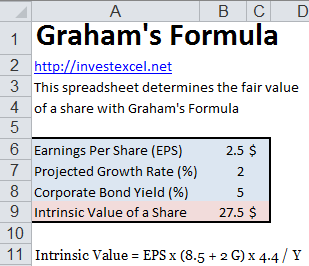# Value Shares with Graham’s Formula

Discover Graham’s formula, a simple method for predicting the fair values of shares, and download a spreadsheet.

Benjamin Graham presented a simple formula to value stock in his 1962 book “The Intelligent Investor”:

Intrinsic Value = EPS x (8.5 + 2g)

The Intrinsic Value is the stock price, EPS is the earnings per share for the last year, and g is the projected growth rate over the next seven to ten years. The 8.5 multiplier is what Graham determined to be the fair PE ratio for a company with no growth (with an earnings yield of 100/8.5% = 11.76%). .

Dividing the intrinsic value by the current stock price gives a number that is often used to screen stocks. If this number is greater than 1, the stock is a good buy. However, if this number is less than 1, the stock is to be avoided.

This formula was developed at a time of  low inflation, with growth being real and tangible and a AAA corporate bond interest of 4.4%. Graham later revised this formula to

Intrinsic Value = EPS x (8.5 + 2g) x 4.4 / Y

where Y is the yield on 20-year corporate AAA rated bonds. The 4.4 references Graham’s desired rate of return.Graham’s Formula is not, however, a magic wand to screen for value stocks. It has several disadvantages.

• The formula only uses EPS to value a company, and does not capture the effect of non-operating liabilities. Consider two companies with with equal profits. The first needs to reinvest less than the second in capital expenditure to maintain the business. Hence the second business has more cash. The first business is certainly far more valuable than the second.
• EPS can be manipulated with creative accounting methods. Instead, a normalized EPS calculated from a 10-year history is sometimes used.
• Often, major changes in the business landscape cause risks that need to be interpreted with human judgement. For example, business selling horses in the early 1900s may not have necessarily forecasted the popularity automobiles.

Benjamin Graham placed several limitations on the use of this formula. He advised that the formula should only be used for financially strong companies, with above-par debt positions. Moreover, Gerald Perritt (in his book “Small Stocks, Big Profits) suggested that Graham’s formula only be used for companies whose

• total asset ratios is less than 60%.
• earnings-to-price ratio (i.e. the reciprocal of the PE ratio) is two times or more greater than the corporate AAA bond yield,
• positive earnings,
• and share price is less than the net working capital per share